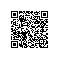# 一道笔试题--求二进制数1的个数

int OneCount0(int i)

{

//将i转化为二进制字符串

String str = i + "";

//然后用StringBuffer类进行按顺序扫面，扫面字符'1'

}

unsigned int OneCount1( unsigned int i )

{

unsigned int count = 0;

unsigned int onetwo;

do

{

onetwo = i%2;

i = i/2; //i = i>>1;

count += onetwo?1:0;

}while ( i != 0 );

return count;

}

unsigned OneCount2(unsigned int i)

{

int j = 0;

while(x)

{

i = i&(i-1);

j ++;

}

return j;

}

unsigned long OneCount3(unsigned int x)

{

x = (x & 0x55555555UL) + ((x >> 1) & 0x55555555UL); //1

x = (x & 0x33333333UL) + ((x >> 2) & 0x33333333UL); //2

x = (x & 0x0f0f0f0fUL) + ((x >> 4) & 0x0f0f0f0fUL); //3

x = (x & 0x00ff00ffUL) + ((x >> 8) & 0x00ff00ffUL); //4

x = (x & 0x0000ffffUL) + ((x >> 16) & 0x0000ffffUL); //5

return x;

}

本文转自 dog250 51CTO博客，原文链接:http://blog.51cto.com/dog250/1274094使用钉钉扫一扫加入圈子
+ 订阅